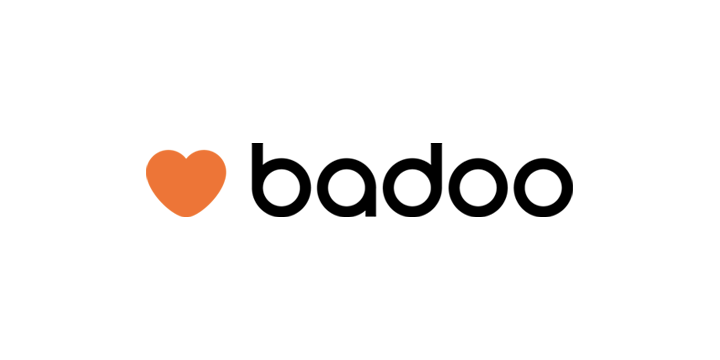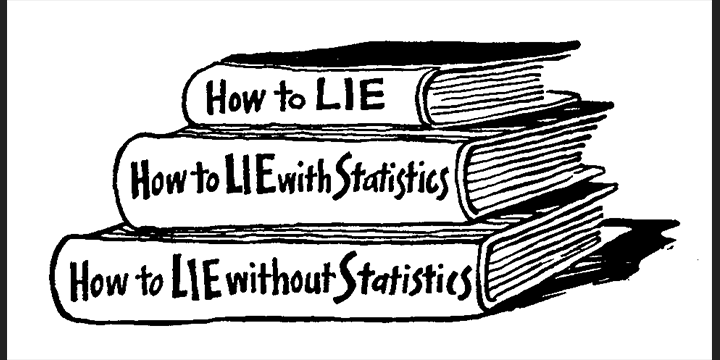This the third and final tutorial in which we build Freehand Drawing for iOS. In this part we’ll improve how the stroke looks and feels.

You can check the previous posts here:

In the last post we did a refactor and implemented undo functionality, thus completing all the features of our small drawing application. Now it is time to improve the look and feel of the drawing itself.

## Lines

We implemented drawing by connecting the dot path the user creates with her finger. We connect the dots by drawing straight lines between touch points: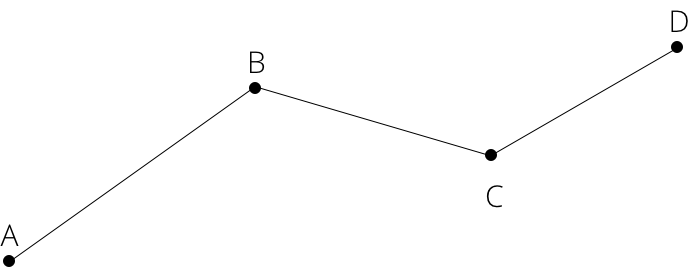This is very simple to implement but the drawing looks artificial and ‘computerish’. This is because a human would never draw this way, unless she stopped and changed direction at every point. This is less noticeable in our implementation because the stroke width is set to a big value. Let’s reduce it and adjust it from there. This is how the stroke looks: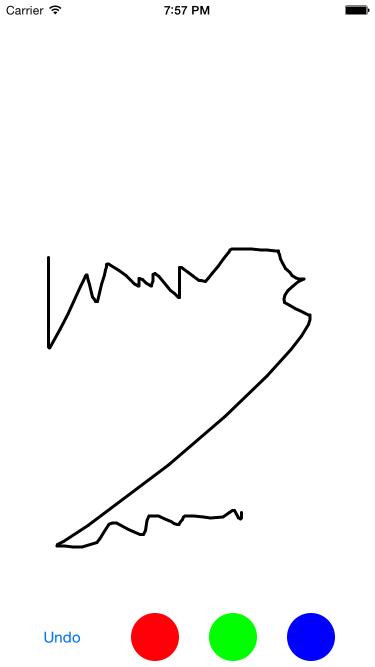## Adding a curve

The first improvement we can make is to add some curvature when we connect the dots. To select how we join the user’s touches we need to satisfy two constraints:

• The points are not known in advance as the user continually touches the screen and creates new points. We draw curves only knowing the previous points.
• We can’t redraw segments when new points are added. This means we are restricted on how we ensure a good curve between segments.

Considering our restrictions, we could try to use the simplest and most popular widely used curve interpolation between two points: Bezier curves.

We won’t use Catmull-Rom spline due to a couple of reasons:

• Catmull requires all points in advance.
• We’d need to implement it ourselves or use a library. We can use Apple’s implementation of Bezier curves in ``` Core Graphics``` framework.

In order to use cubic bezier curve, we need to specify two target points and two control points: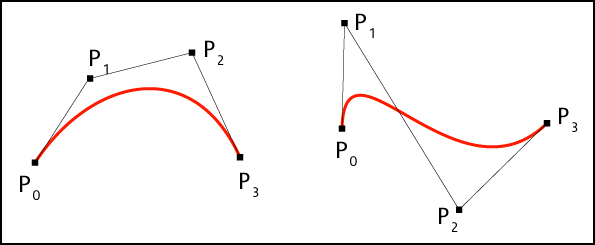Similarly, a quadratic bezier curve is specified by two target points, but only one control point: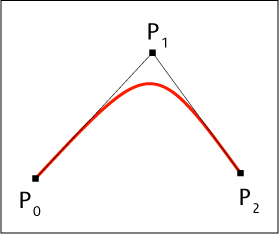When using Bezier paths, the choice of control points is vital, as they define the curvature and tangent direction on the target points. For now we’ll use quadratic Bezier because it only needs one control point, so it's easier to calculate. We can adopt a cubic Bezier path later if the result is not satisfactory.

### Curves with Bezier splines

We already know that choosing a good control point is key to ensuring our curves are smooth and continuous. If we were to take the control point as the midpoint between two touches, even if it seemed that it would work nicely, the curve would break at every point, because the tangents at the target points wouldn’t match: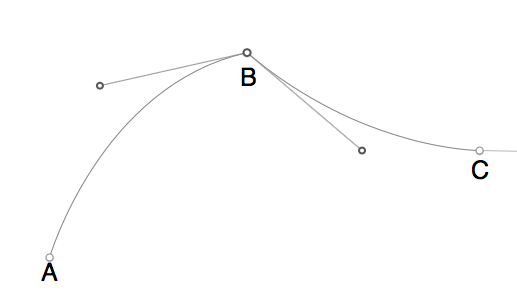We can use a more sophisticated but still simple choice of control point. We’ll need three touch points. The control point will be the second touch. We will then use midpoints between first and second, second and third, as the target points. The line will not go exactly through the user’s touches, but the difference is small enough not to be noticed. Most importantly, the tangent is preserved at the target points, thus keeping a smooth curve throughout the user touches: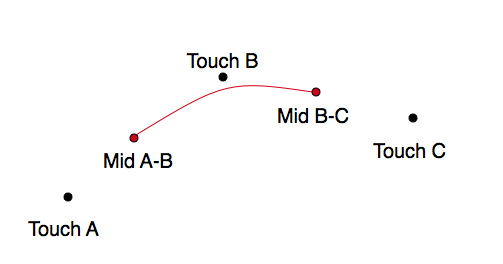We encourage you to check this excellent tutorial, which explains this choice of control points in depth. Now let’s modify our code to support drawing curves.

First we need to modify our ``` LineDrawCommand```. When the user only moves the finger producing two touches, we can’t draw more than a straight line, as we don’t have previous points data. Afterwards, to trace a quadratic bezier using the midpoints we will need three touches.

You can see all the changes here.

Let's start by explicitly having a model of a segment in our code to increase readability:

``````

struct Segment {
let a: CGPoint
let b: CGPoint

var midPoint: CGPoint {
return CGPoint(x: (a.x + b.x) / 2, y: (a.y + b.y) / 2)
}
}``````

We will initialise our draw command with one segment, and optionally a second one, to form the three required touch points. When all points are provided, the command will draw a curve. If only two points are provided, the command will draw a line instead.

``````
struct LineDrawCommand : DrawCommand {
let current: Segment
let previous: Segment?

let width: CGFloat
let color: UIColor

// MARK: DrawCommand

func execute(canvas: Canvas) {
self.configure(canvas)

if let previous = self.previous {
} else {
self.drawLine(canvas)
}
}

private func configure(canvas: Canvas) {
CGContextSetStrokeColorWithColor(canvas.context, self.color.CGColor)
CGContextSetLineWidth(canvas.context, self.width)
CGContextSetLineCap(canvas.context, kCGLineCapRound)
}

private func drawLine(canvas: Canvas) {
CGContextMoveToPoint(canvas.context, self.current.a.x, self.current.a.y)
CGContextStrokePath(canvas.context)
}

private func drawQuadraticCurve(canvas: Canvas) {
if let previousMid = self.previous?.midPoint {
let currentMid = self.current.midPoint

CGContextMoveToPoint(canvas.context, previousMid.x, previousMid.y)
CGContextStrokePath(canvas.context)
}
}
}``````

The last change is to our ``` DrawController```. It needs to use the changed LineDrawCommand, and retain the state of previous segments as the user continues moving her finger.

``````
// In DrawController.swift
private func continueAtPoint(point: CGPoint) {
let segment = Segment(a: self.lastPoint, b: point)

let lineCommand = LineDrawCommand(current: segment, previous: lastSegment, width: self.width, color: self.color)

self.canvas.executeCommands([lineCommand])

self.lastPoint = point
self.lastSegment = segment
}

// Other minor cleanup necessary, please refer to the repository``````

If you run and test it now the stroke is much less blocky: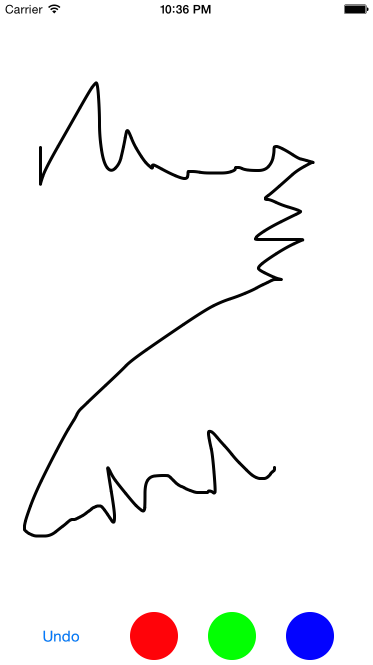If you would like to improve the stroke even further, you can try adopting cubic bezier paths. To use those you’ll need an additional control point.

## Changing the stroke width

Up until now, our stroke has been uniform in width. This is practical and easy to implement, but we can add a nice touch to give our feature a distinctive and playful look. We’ll change the width of the stroke depending on how fast the user is moving her finger.

We want to remind the user of something from the real world without emulating it perfectly, as we are not building a drawing application. We can even exaggerate the effect a bit to make it more interesting.

As we are not drawing triangles, but just using higher level line drawing commands, we only have control of the width of one segment. So we will need to add width to our segment structure.

The width of the current segment is inversely proportional to the speed of the movement. This means the faster the user moves the finger the thinner the stroke will be. The velocity is supplied by the gesture recogniser, so changing our ``` DrawController``` to use variable width is very easy. We just need to use that velocity, and we can isolate the actual calculation of the width change in a free function.

You can also see these changes here.

``````
func modulatedWidth(width: CGFloat, velocity: CGPoint) -> CGFloat {
let velocityAdjustement: CGFloat = 600.0 // Trial and error constant
let speed = velocity.length() / velocityAdjustement

let modulated = width / speed
return modulated
}

extension CGPoint {
func length() -> CGFloat {
return sqrt((self.x*self.x) + (self.y*self.y))
}
}``````

This code will modulate the width but in a very strange way: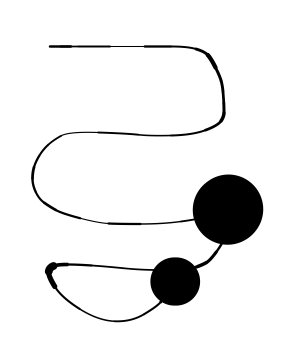There are two problems with our simple width modulation:

1. Speed can change a great deal between touches.
2. We don't limit the width to between a minimum and a maximum.

To solve the first problem we’ll keep track of the previous speed and give more weight to it when calculating the modulated width. This will work for cases when the user makes sudden changes of speed, so the the actual change of width will be more gradual.

For the second problem we’ll just limit the output width to between a maximum and a minimum value.

These changes are here.

``````
func modulatedWidth(width: CGFloat, velocity: CGPoint, previousVelocity: CGPoint, previousWidth: CGFloat) -> CGFloat {
let velocityAdjustement: CGFloat = 600.0
let speed = velocity.length() / velocityAdjustement
let previousSpeed = previousVelocity.length() / velocityAdjustement

let modulated = width / (0.6 * speed + 0.4 * previousSpeed)
let limited = clamp(modulated, 0.75 * previousWidth, 1.25 * previousWidth)
let final = clamp(limited, 0.2*width, width)

return final
}

extension CGPoint {
func length() -> CGFloat {
return sqrt((self.x*self.x) + (self.y*self.y))
}
}

func clamp<T: Comparable>(value: T, min: T, max: T) -> T {
if (value < min) {
return min
}

if (value > max) {
return max’
}

return value
}

// Additional cleanup and setup in draw controller to keep
// track of previous width and previous velocity. See repository.``````

The parameters are a bit extreme to see the difference, but this is how the stroke looks with this code: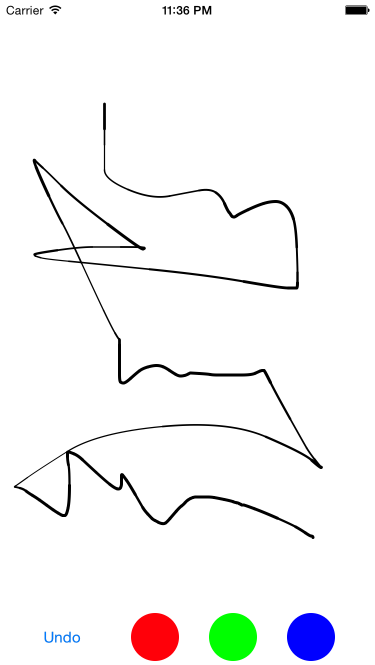Now we’re getting the impression of drawing with a dip pen. You’ll need to tweak the constants to your liking depending on how subtle you want it to be. We don't want to simulate a real stroke with ink, but rather to give a playful and more realistic feel for the user.

## Conclusion

We’ve improved the stroke of our small drawing application by connecting the dots using more than just straight lines. We’ve also achieved a more realistic and playful feel by changing the width of the stroke depending on the speed of user touches.

During the course of these tutorials we’ve seen the kind of technical challenges a developer may be faced with, and we’ve evolved our code by refactoring and redesigning as our requirements change.

The repository with all the code can be found here.